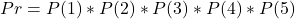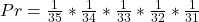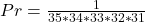## In a certain lottery, five different numbers between 1 and 35 inclusive are drawn. These are the winning numbers. To win the lottery, a pers

Question

In a certain lottery, five different numbers between 1 and 35 inclusive are drawn. These are the winning numbers. To win the lottery, a person must select the correct 5 numbers in the same order in which they were drawn. What is the probability of winning

in progress 0
2 months 2021-07-22T08:56:55+00:00 1 Answers 3 views 0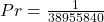Step-by-step explanation:

Given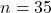—- 1 to 35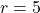— selection

Required

The probability of winning

The probability of getting the first number correctly is: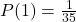At this point, the remaining numbers are 34

So, the second selection has the following probability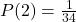Following the above sequence, we have: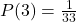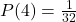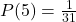So, the required probability is: